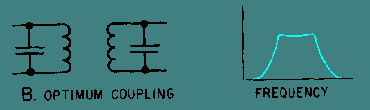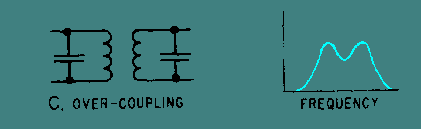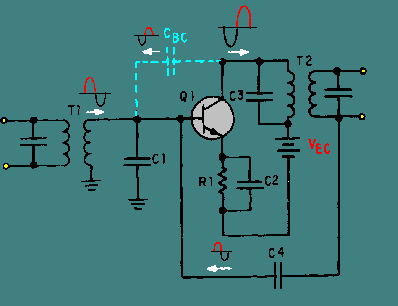rf amplifiers; for example, transformer coupling uses fewer components than capacitive coupling.">Custom SearchFigure 2-14 and the other circuits you have been shown use capacitors to couple the signal in to and out of the circuit (C1 and C4 in figure 2-14). As you remember from chapter 1, there are also other methods of coupling signals from one stage to another. Transformer coupling is the most common method used to couple rf amplifiers. Transformer coupling has many advantages over RC coupling for rf amplifiers; for example, transformer coupling uses fewer components than capacitive coupling. It can also provide a means of increasing the gain of the stage by using a step-up transformer for voltage gain. If a current gain is required, a step-down transformer can be used. You should also remember that the primary and secondary windings of a transformer are inductors. With these factors in mind, an rf amplifier could be constructed like the one shown in figure 2-15. Figure 2-15. - Transformer-coupled rf amplifier.In this circuit, the secondary of T1 and capacitor C1 form a tuned circuit which is the input-signal-developing impedance. The primary of T2 and capacitor C2 are a tuned circuit which acts as the output impedance of Q1. (Both T1 and T2 must be rf transformers in order to operate at rf frequencies.) The input signal applied to the primary of T1 could come from the previous stage or from some input device, such as a receiving antenna. In either case, the input device would have a capacitor connected across a coil to form a tuned circuit. In the same way, the secondary of T2 represents the output of this circuit. A capacitor connected across the secondary of T2 would form a parallel LC network. This network could act as the input-signal-developing impedance for the next stage, or the network could represent some type of output device, such as a transmitting antenna. The tuned circuits formed by the transformer and capacitors may not have the bandwidth required for the amplifier. In other words, the bandwidth of the tuned circuit may be too "narrow" for the requirements of the amplifier. (For example, the rf amplifiers used in television receivers usually require a bandwidth of 6 MHz.) One way of "broadening" the bandpass of a tuned circuit is to use a swamping resistor. This is similar to the use of the swamping resistor that was shown with the series peaking coil in a video amplifier. A swamping resistor connected in parallel with the tuned circuit will cause a much broader bandpass. (This technique and the theory behind it are discussed in more detail in NEETS, module 9.) Another technique used to broaden the bandpass involves the amount of coupling in the transformers. For transformers, the term "coupling" refers to the amount of energy transferred from the primary to the secondary of the transformer. This depends upon the number of flux lines from the primary that intersect, or cut, the secondary. When more flux lines cut the secondary, more energy is transferred. Coupling is mainly a function of the space between the primary and secondary windings. A transformer can be loosely coupled (having little transfer of energy), optimumly coupled (just the right amount of energy transferred), or overcoupled (to the point that the flux lines of primary and secondary windings interfere with each other). Figure 2-16, (view A) (view B) (view C), shows the effect of coupling on frequency response when parallel LC circuits are made from the primary and secondary windings of transformers. Figure 2-16A. - Effect of coupling on Frequency response. LOOSE COUPLINGFigure 2-16B. - Effect of coupling on Frequency response. OPTIMUM COUPLINGFigure 2-16C. - Effect of coupling on Frequency response. OVER-COUPLINGIn view (A) the transformer is loosely coupled; the Frequency response curve shows a narrow bandwidth. In view (B) the transformer has optimum coupling; the bandwidth is wider and the curve is relatively flat. In view (C) the transformer is overcoupled; the Frequency response curve shows a broad bandpass, but the curve "dips" in the middle showing that these frequencies are not developed as well as others in the bandwidth. Optimum coupling will usually provide the necessary bandpass for the frequency-determining network (and therefore the rf amplifier). For some uses, such as rf amplifiers in a television receiver, the bandpass available from optimum coupling is not wide enough. In these cases, a swamping resistor (as mentioned earlier) will be used with the optimum coupling to broaden the bandpass. COMPENSATION OF RF AMPLIFIERS Now you have been shown the way in which an rf amplifier is configured to amplify a band of frequencies and the way in which an rf amplifier can be "tuned" for a particular band of frequencies. You have also seen some ways in which the bandpass of an rf amplifier can be adjusted. However, the frequencies at which rf amplifiers operate are so high that certain problems exist. One of these problems is the losses that can occur in a transformer at these high frequencies. Another problem is with interelectrode capacitance in the transistor. The process of overcoming these problems is known as COMPENSATION. Transformers in RF Amplifiers As you recall from NEETS, module 1, the losses in a transformer are classified as copper loss, eddy-current loss, and hysteresis loss. Copper loss is not affected by frequency, as it depends upon the resistance of the winding and the current through the winding. Similarly, eddy-current loss is mostly a function of induced voltage rather than the frequency of that voltage. Hysteresis loss, however, increases as frequency increases. Hysteresis loss is caused by the realignment of the magnetic domains in the core of the transformer each time the polarity of the magnetic field changes. As the frequency of the a.c. increases, the number of shifts in the magnetic field also increases (two shifts for each cycle of a.c.); therefore, the "molecular friction" increases and the hysteresis loss is greater. This increase in hysteresis loss causes the efficiency of the transformer (and therefore the amplifier) to decrease. The energy that goes into hysteresis loss is taken away from energy that could go into the signal. RF TRANSFORMERS, specially designed for use with rf, are used to correct the problem of excessive hysteresis loss in the transformer of an rf amplifier. The windings of rf transformers are wound onto a tube of nonmagnetic material and the core is either powdered iron or air. These types of cores also reduce eddy-current loss. Neutralization of RF Amplifiers The problem of interelectrode capacitance in the transistor of an rf amplifier is solved by NEUTRALIZATION. Neutralization is the process of counteracting or "neutralizing" the effects of interelectrode capacitance. Figure 2-17 shows the effect of the base-to-collector interelectrode capacitance in an rf amplifier. The "phantom" capacitor (CBC) represents the interelectrode capacitance between the base and the collector of Q1. This is the interelectrode capacitance that has the most effect in an rf amplifier. As you can see, CBC causes a degenerative (negative) feedback which decreases the gain of the amplifier. (There are some special cases in which CBC can cause regenerative (positive) feedback. In this case, the technique described below will provide negative feedback which will accomplish the neutralization of the amplifier.) Figure 2-17. - Interelectrode capacitance in an rf amplifier.As you may recall, unwanted degenerative feedback can be counteracted (neutralized) by using positive feedback. This is exactly what is done to neutralize an rf amplifier. Positive feedback is accomplished by the use of a feedback capacitor. This capacitor must feed back a signal that is in phase with the signal on the base of Q1. One method of doing this is shown in figure 2-18. Figure 2-18. - Neutralized rf amplifier.In figure 2-18, a feedback capacitor (C4) has been added to neutralize the amplifier. This solves the problem of unwanted degenerative feedback. Except for capacitor C4, this circuit is identical to the circuit shown in figure 2-17. (When CBC causes regenerative feedback, C4 will still neutralize the amplifier. This is true because C4 always provides a feedback signal which is 180 degrees out of phase with the feedback signal caused by CBC.) Q.25 What is the most common form of coupling for an rf amplifier?Q.26 What are two advantages of this type of coupling?Q.27 If current gain is required from an rf amplifier, what type of component should be used as an output coupling element?Q.28 What problem is caused in an rf amplifier by a loosely coupled transformer?Q.29 How is this problem corrected?Q.30 What problem is caused by overcoupling in a transformer?Q.31 What method provides the widest bandpass?Q.32 What two methods are used to compensate for the problems that cause low gain in an rf amplifier?Q.33 What type of feedback is usually caused by the base-to-collector interelectrode capacitance?Q.34 How is this compensated for?Privacy Statement - Copyright Information. - Contact Us